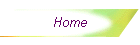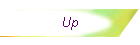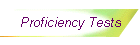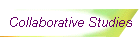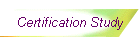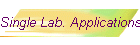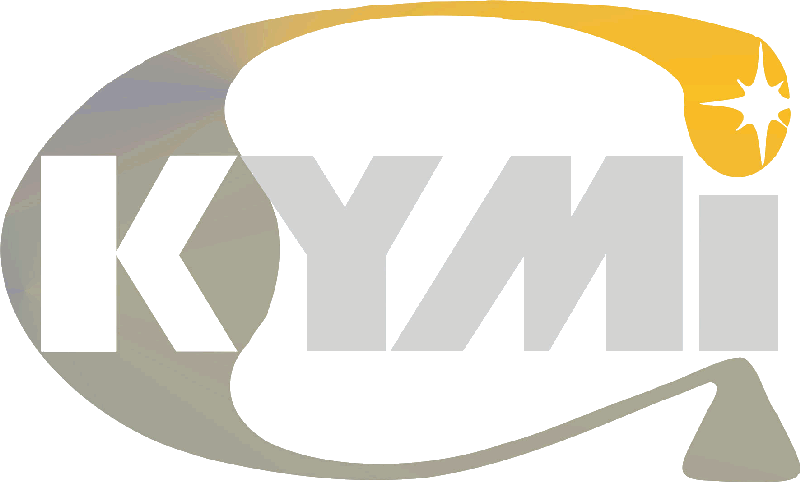## Single Laboratory Applications

InterLab2.0 is not intended for use only in interlaboratory work. It contains tools and techniques, which are very useful for method validation in single laboratories.

Here follows an example. A geochemical laboratory wanted to test different methods for analysing base metals in soils and sediments. It had in routine use a method for geochemical analyses based on the partial digestion of the sample in aqua regia, followed by analysis in an ICP-AES instrument. It wanted to test the feasibility of producing good results with X Ray Fluorescence Spectrometry (XRF). For the sake of comparing those two methods, a reference method was used (here called the ‘ore method’), with complete digestion of the sample in a multi-acid solution, and completion of the work with atomic absorption or ICP-AES, depending on the chemical element.

Theoretically, it was already known that the ore-method should produce higher results than the geochemical one, as the aqua regia used was expected not to put into solution all the metals with a 100% recovery. Even so, the lab manager wanted to have information on the degree to which each metal would be dissolved, by comparing results of the geochemical method with the ore-method. And knowing this, evaluate if the XRF method was good.

Sixteen samples were selected, and run 20 times by each method, in twenty different days, in order to provide replicates in repeatability conditions. The analyses performed were for Co, Cr, Cu, Fe, Mn, Mo, Ni, Pb and Zn. A first evaluation of the results can be done using Mandel’s h and k consistency statistics. For example, Ni results are shown. This plot shows that the ore method is systematically higher than the other methods. This would seem to indicate that the geochemical method is not capable of solubilising all the Ni present in the sample, while the XRF method may have some calibration problem.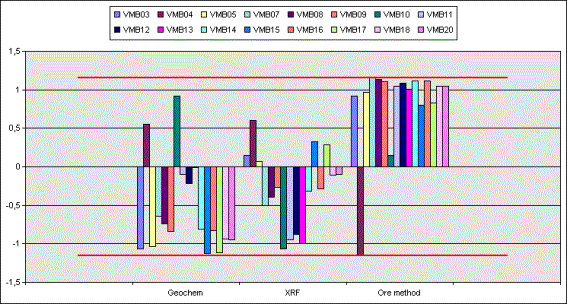Looking at the table for Fe analysis, with the methods means and standard deviations, marked by the outliers tests, one can see that in general the geochemical method is much less precise than the other two methods (a lot of std. deviation values are marked with a red K, which means that it is a Mandel k’s outlier).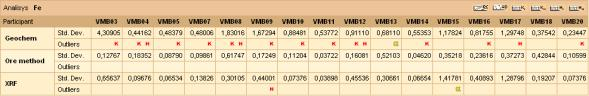Confirming this with a graphic,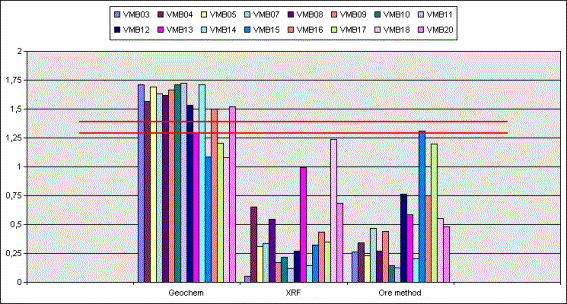The precision obtained for each method can be obtained directly from the screen with  the precision curves

Evaluation of the relative bias of the Fe methods, considering the ore-method as the one with true results for evaluating the geochemical method, gives the following analysis: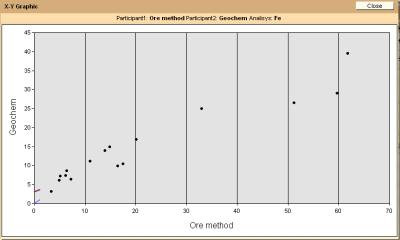It seems that the geochemical method correlates well with the ore method up to a certain concentration (near 40% Fe). After that, it shows a sort of ‘saturation’, and it seems that the method beyond this point is not capable of putting into solution the Fe atoms anymore. This could be caused by the fact that about 40g of material are attacked by 10 mL of aqua regia. That concentration may be the one where all the acid is consumed and is not able of extracting anymore metal into solution.

Bias evaluation by regression analysis gives the hypothesis test illustrated below, where it may be seen that both fixed and proportional biases are present. The fixed bias is of the order of 3% Fe, and the proportional one, about 40% relative lower. This means that the Geochem method finds results about 40% below those provided by the Ore method, plus a constant bias of 3%. Of course, this analysis could be improved if the partition in Fe concentration is considered. As the method seems to behave well up to a certain concentration, and then it begins to saturate, additional evaluations could be done separating the data below and above the cut-off point in Fe concentration.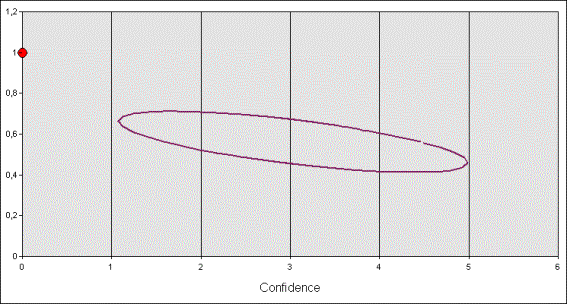In conclusion, InterLab2.0 is a powerful tool for evaluating methods developed in-house, which otherwise would be extremely time consuming to evaluate using only the usual tools the chemists are used to, such as spreadsheets, or even standard statistical packages.